# Triangle Trigonometry Lesson Plans Chapter Exam

Exam Instructions:

Choose your answers to the questions and click 'Next' to see the next set of questions. You can skip questions if you would like and come back to them later with the yellow "Go To First Skipped Question" button. When you have completed the practice exam, a green submit button will appear. Click it to see your results. Good luck!

### Page 1

#### Question 1 1. Find the value of x, rounded to the nearest degree.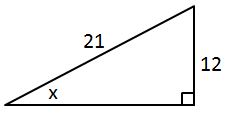#### Question 2 2. In the pictured triangle, what ratio represents the cosine of theta?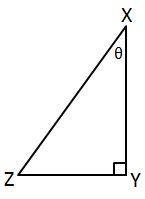#### Question 4 4. Find the value of x, rounded to the nearest tenth.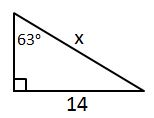### Page 2

#### Question 7 7. Find the value of x, rounded to the nearest degree.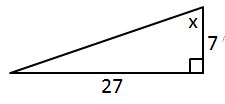#### Question 8 8. Find the value of x, rounded to the nearest tenth.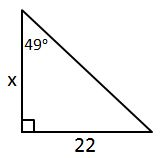#### Question 9 9. What is x?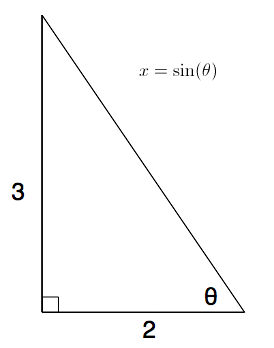### Page 3

#### Question 12 12. What is z in terms of x and y?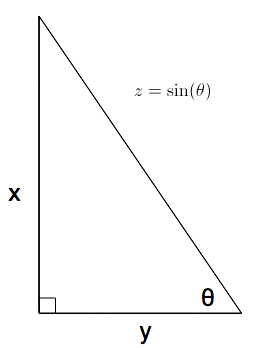#### Question 13 13. What is x?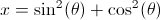#### Question 15 15. Jerry wants to buy a TV online, but the ad for the TV he wants to buy does not say what the screen size is. He knows that TV screens are measured diagonally. This image shows the length and width of the screen, so he decides to use the Pythagorean Theorem to determine the diagonal screen size. What is the screen size of the TV that Jerry wants to buy (to the nearest inch)?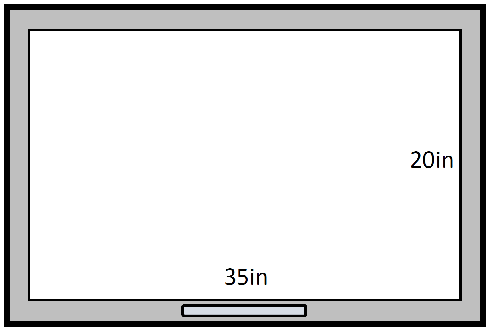### Page 4

#### Question 16 16. Which of the following is true?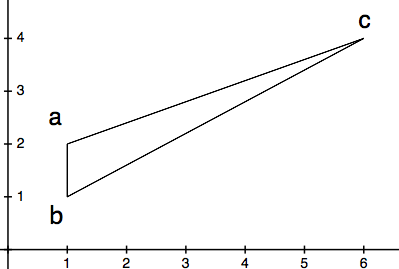### Page 5

#### Question 21 21. Find the shortest distance between a and b by drawing a line connecting them and using the Pythagorean Theorem.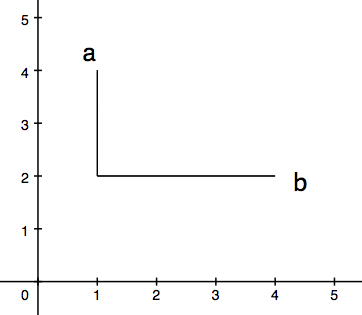#### Question 23 23. What is z in terms of x?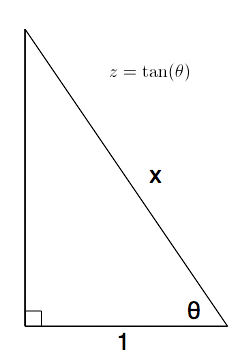#### Question 24 24. Find the value of x, rounded to the nearest tenth.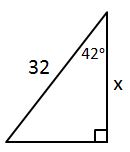#### Triangle Trigonometry Lesson Plans Chapter Exam Instructions

Choose your answers to the questions and click 'Next' to see the next set of questions. You can skip questions if you would like and come back to them later with the yellow "Go To First Skipped Question" button. When you have completed the practice exam, a green submit button will appear. Click it to see your results. Good luck!

Support